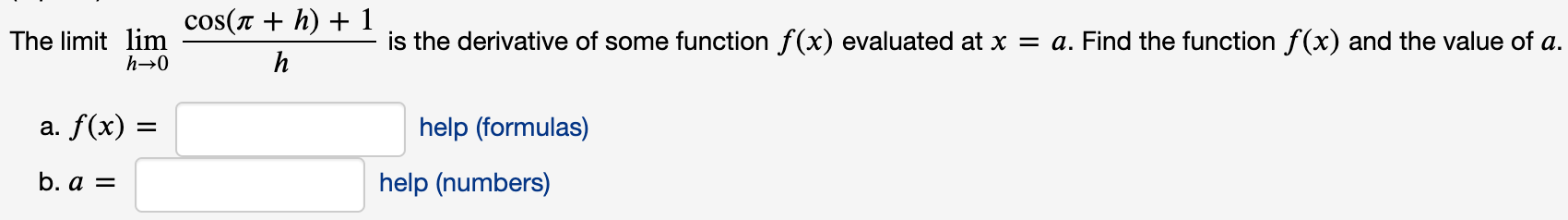# cos(r + h) + 1 The limit lim h→0 is the derivative of some function f(x) evaluated at x = a. Find the function f(x) and the value of a. h a. f(x) = help (formulas) %D b. a = help (numbers)

Questionhelp_outlineImage Transcriptionclosecos(r + h) + 1 The limit lim h→0 is the derivative of some function f(x) evaluated at x = a. Find the function f(x) and the value of a. h a. f(x) = help (formulas) %D b. a = help (numbers) fullscreen

### Want to see this answer and more?

Experts are waiting 24/7 to provide step-by-step solutions in as fast as 30 minutes!*

*Response times may vary by subject and question complexity. Median response time is 34 minutes for paid subscribers and may be longer for promotional offers.
Tagged in
Math
Calculus

### Other